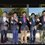# IPhO OCSC Preparation

I somehow predicted that there was a certainty that difficult and quite lengthy calculative problems would be asked in this year's OCSC.

In 2017 the paper was only based on calculation. Problems were only lengthy calculations.There were 2 theory problems and 2 practicals. Where 3-4 experiments are asked, it was 5-6 at 2017 OCSC. Experiments were 5 hours long. There was an experiment sufrace tension of water drops. I recall, we had to count 10000-12000 water drops. :P

I felt 2018 had better problems than 2017. It was trickier and quite interesting. There was a mechanical black box experiment. Other practicals were Wind chimes, Characteristics of LED, electrical transmissions of an Inductor. I solely wished to propose problems for 2019 OCSC, but didn't propose any problems this year's OCSC :P.

Hope all of you, enjoyed the problems of 2019 OCSC. They've made the theory easy, removed the super difficult parts of the proposed problems. There was one from past INPhO, the official solution by HBCSE is worng, none of them got actually able to ace that. Basically, experiments made the real difference. Those juniors who couldn't make it to the team, my best wishes to them. Do your best, may the force be with you.

Note- The practicals aren't repeated in every year. But there is always an experiment, where would be a pendulum and black box.

# OCSCNote by Pawan Goyal
1 month, 1 week ago

MarkdownAppears as
*italics* or _italics_ italics
**bold** or __bold__ bold
- bulleted- list
• bulleted
• list
1. numbered2. list
1. numbered
2. list
Note: you must add a full line of space before and after lists for them to show up correctly
paragraph 1paragraph 2

paragraph 1

paragraph 2

[example link](https://brilliant.org)example link
> This is a quote
This is a quote
    # I indented these lines
# 4 spaces, and now they show
# up as a code block.

print "hello world"
# I indented these lines
# 4 spaces, and now they show
# up as a code block.

print "hello world"
MathAppears as
Remember to wrap math in $ ... $ or $ ... $ to ensure proper formatting.
2 \times 3 $2 \times 3$
2^{34} $2^{34}$
a_{i-1} $a_{i-1}$
\frac{2}{3} $\frac{2}{3}$
\sqrt{2} $\sqrt{2}$
\sum_{i=1}^3 $\sum_{i=1}^3$
\sin \theta $\sin \theta$
\boxed{123} $\boxed{123}$